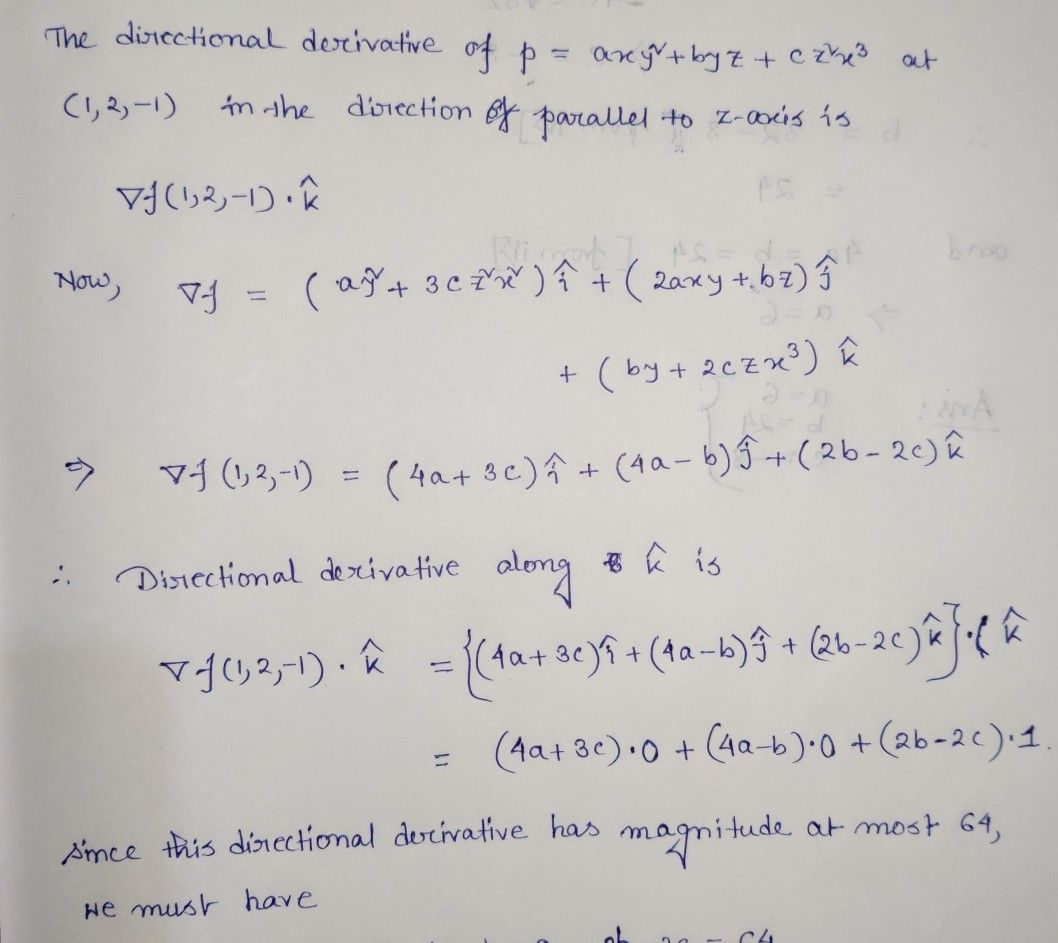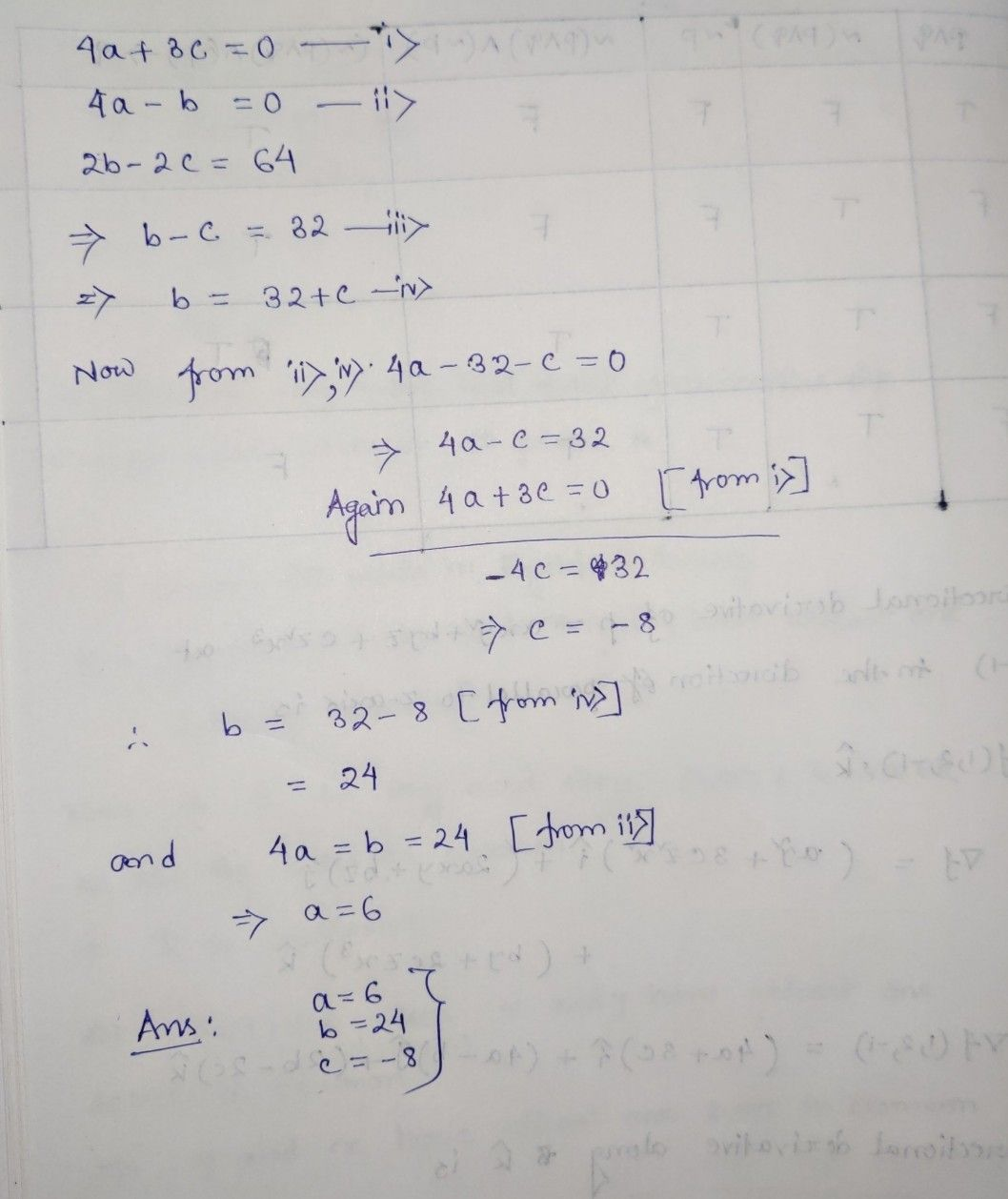Symbol
Problem$8.$ a. Find the values of $a,$ $b,$ $C$ so that the directional derivative of $p=axy^{2}+byz+cz^{2}x^{3}$ at $t\left(1,2,-1\right)$ has maximum magnitude $64$ in the direction parallel to $7-a\times 1s$ $7M$
Calculus
Search count: 110
SolutionQanda teacher - LubdhStudent
Tq for the solutionQanda teacher - Lubdh
welcome